Courses

# Homomorphism, Group Theory Mathematics Notes | EduRev

## Algebra for IIT JAM Mathematics

Created by: Veda Institute

## Mathematics : Homomorphism, Group Theory Mathematics Notes | EduRev

The document Homomorphism, Group Theory Mathematics Notes | EduRev is a part of the Mathematics Course Algebra for IIT JAM Mathematics.
All you need of Mathematics at this link: Mathematics

Homomorphisms are the maps between algebraic objects. There are two main types: group homomorphisms and ring homomorphisms. (Other examples include vector spacehomomorphisms, which are generally called linear maps, as well as homomorphisms of modules and homomorphisms of algebras.)

Generally speaking, a homomorphism between two algebraic objects A, B is a function f : A → B which preserves the algebraic structure on A and B That is, if elements in A satisfy some algebraic equation involving addition or multiplication, their images in B satisfy the same algebraic equation. The details of the definitions of homomorphisms in various contexts depend on the algebraic structures of A and B.

 EXAMPLEIf the operations on A and B are both addition, then the homomorphism condition is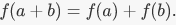If A and B are both rings, with addition and multiplication, there is also a multiplicative condition: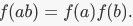A bijective homomorphism is called an isomorphism. An isomorphism between two algebraic objects  and  identifies them with each other; they are, in an algebraic sense, the same object (possibly written in two different ways). The most common use of homomorphisms in abstract algebra is via the three so-called isomorphism theorems, which allow for the identification of certain quotient objects with certain other subobjects (subgroups, subrings, etc.)

The study of the interplay between algebraic objects is fundamental in the study of algebra. The existence and properties of homomorphisms from one algebraic object to another give a rich depth of information about the objects and their relationship. Many important concepts in abstract algebra, such as

• the integers modulo n

• a prime ideal in a ring

• the sign of a permutation,

can be naturally considered as (respectively) the image of a homomorphism, the kernel of a homomorphism, or the homomorphism itself.

Definitions and Examples

 Let A and B be groups, with operations given by  ºA and ºB respectively. A group homomorphism f : A → B is a function f such that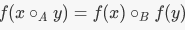for all x,y ∈ A.

 DEFINATIONLet R and S be rings, with operations + and . (this is a slight abuse of notation, but the formulas below are more unwieldy with subscripts on the operations). A ring homomorphism f : R → S is a function f such that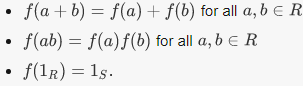(In this wiki, "ring" means "ring with unity"; a homomorphism of rings is defined in the same way, but without the third condition.)

In both cases, a homomorphism is called an isomorphism if it is bijective.

 EXAMPLEShow that if f : R → S is a ring homomorphism, f(0R) = os.Note that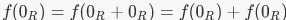by the homomorphism property. Since f(0R) has an additive inverse in S, we can add it to both sides of this equation to get 0S =  f(0R).

 EXAMPLE1. For any groups G and H, there is a trivial homomorphis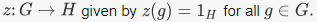2. Let  be a positive integer. The function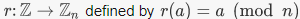is a ring homomorphism (and as such, it is a homomorphism of additive groups).3. Define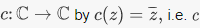is complex conjugation. Then c is a homomorphism from  C to itself. It is clearly a bijection, so it is in fact an isomorphism from C to itself.4. Let R be a subring of S, and pick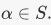Then there is an evaluation homomorphism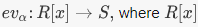is the ring of polynomials with coefficients in R.It is given by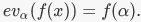5. The map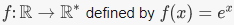is a group homomorphism. Note that R is an additive group and R* the set of nonzero real numbers, is a multiplicative group. The verification that f is a group homomorphism is precisely the law of exponents: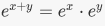6. Let Sn be the symmetric group on n letters. There is a unique nontrivial group homomorphism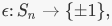the latter being a group under multiplication. The value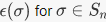is called the sign of σ, and is important in many applications, including one definition of the determinant of a matrix.

Kernel and Image

Any homomorphism f : A → B has two objects associated to it: the kernel, which is a subset of A, and the image, which is a subset of B.

 DEFINATION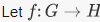be a group homomorphism. The kernel of f, ker (f), is the subset of G consisting of elements G such that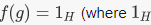is the group identity element).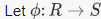be a ring homomorphism. The kernel of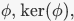is the subset of  R consisting of elements R such that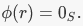For further exploration of the kernel in the setting of vector spaces, see the wiki.

The kernel of a homomorphism is an important object, in both group and ring theory. The following theorem identifies what kind of object it is:

 EXAMPLEContinuing the six examples above:1. If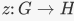is the trivial homomorphism, then ker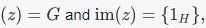the trivial subgroup of  H2. The kernel of reduction mod n is the ideal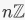consisting of multiples of n. The image is all of Zn; reduction mod n is surjective.3. The kernel of complex conjugation is {0}, the trivial ideal of C (Note that 0 is always in the kernel of a ring homomorphism, by the above example.) The image is all of C.4. The kernel of evaluation at α is the set of polynomials with coefficients in R which vanish at α. This ideal is not always easy to determine, depending on the nature of R and S. To take a common example, suppose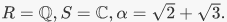Which polynomials with rational coefficients vanish on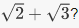(See the algebraic number theory wiki for an answer.)The image of evaluation at α is a ring called R[α], which is a subring of S consisting of polynomials in α with coefficients in R.5. The kernel of exponentiation is the set of elements which map to the identity element of R*, which is 1 So the kernel is {0}. And the image of exponentiation is the subgroup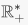of positive real numbers.6. The kernel of the sign homomorphism is known as the alternating group An. It is an important subgroup of Sn which furnishes examples of simple groups for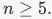The image of the sign homomorphism is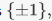since the sign is a nontrivial map, so it takes on both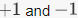for certain permutations.

Properties of Homomorphisms

• Composition: The composition of homomorphisms is a homomorphism. That is, if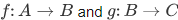are homomorphisms, then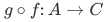is a homomorphism as well.
• Isomorphisms: If f is an isomorphism, which is a bijective homomorphism, then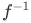is also a homomorphism. (Compare with homeomorphism, a similar concept in topology, which is a continuous function with a continuous inverse; a bijective continuous function does not necessarily have a continuous inverse.)
• Injectivity and the kernel: A group homomorphism f is injective if and only if its kernel  ker(f) equals {1}, where denotes the identity element of the domain. A ring homomorphism is injective if and only if its kernel equals {0} where 0 denotes the additive identity of the domain.
• Field homomorphisms: If R is a field and S is not the zero ring, then any homomorphism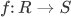is injective. (Proof: the kernel is an ideal, and the only ideals in a  field are the entire field and the zero ideal. Since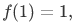it must be the latter.)
Offer running on EduRev: Apply code STAYHOME200 to get INR 200 off on our premium plan EduRev Infinity!

58 docs

,

,

,

,

,

,

,

,

,

,

,

,

,

,

,

,

,

,

,

,

,

,

,

,

;